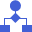This site uses cookies. By continuing to browse the ConceptDraw site you are agreeing to our Use of Site Cookies.

# Solving quadratic equation algorithm - Flowchart

## Solving quadratic equation algorithm - Flowchart

"In elementary algebra, a quadratic equation (from the Latin quadratus for "square") is any equation having the form
ax^2+bx+c=0
where x represents an unknown, and a, b, and c are constants with a not equal to 0. If a = 0, then the equation is linear, not quadratic. The constants a, b, and c are called, respectively, the quadratic coefficient, the linear coefficient and the constant or free term.
Because the quadratic equation involves only one unknown, it is called "univariate". The quadratic equation only contains powers of x that are non-negative integers, and therefore it is a polynomial equation, and in particular it is a second degree polynomial equation since the greatest power is two.
Quadratic equations can be solved by a process known in American English as factoring and in other varieties of English as factorising, by completing the square, by using the quadratic formula, or by graphing." [Quadratic equation. Wikipedia]
The flowchart example "Solving quadratic equation algorithm" was created using the ConceptDraw PRO diagramming and vector drawing software extended with the Mathematics solution from the Science and Education area of ConceptDraw Solution Park. Read more
Used Solutions

## Top 5 Android Flow Chart Apps

For many years CS Odessa corporation has been developing the multifunctional vector diagramming software with supporting sophisticated drawing tools for vector diagramming and design on Macintosh and PC with Windows installed. We feel that our considerable experience gives us an opportunity to look objectively at the current offerings and to offer you our opinion about the main contenders and pretenders. Would you like to know the details about what is going on in the world of Flow Chart drawing applications that support Android? The Top 5 of drawing applications in this space rapidly changes, consequently it is significant understatement to call this application space dynamic. Now, the list of Top 5 popular flowchart makers for Android includes LLNL Flow Charts, Army Flow Charts, DroidDia prime, Note Droid, DroidDia PRO unlocker. These listed business graphics applications are recognized by many experts the most convenient, powerful, and successful for use when drawing on Android devices. Read more

## Euclidean algorithm - Flowchart

"In mathematics, the Euclidean algorithm, or Euclid's algorithm, is a method for computing the greatest common divisor (GCD) of two (usually positive) integers, also known as the greatest common factor (GCF) or highest common factor (HCF). ...
The GCD of two positive integers is the largest integer that divides both of them without leaving a remainder (the GCD of two integers in general is defined in a more subtle way).
In its simplest form, Euclid's algorithm starts with a pair of positive integers, and forms a new pair that consists of the smaller number and the difference between the larger and smaller numbers. The process repeats until the numbers in the pair are equal. That number then is the greatest common divisor of the original pair of integers.
The main principle is that the GCD does not change if the smaller number is subtracted from the larger number. ... Since the larger of the two numbers is reduced, repeating this process gives successively smaller numbers, so this repetition will necessarily stop sooner or later - when the numbers are equal (if the process is attempted once more, one of the numbers will become 0)." [Euclidean algorithm. Wikipedia]
The flowchart example "Euclidean algorithm" was created using the ConceptDraw PRO diagramming and vector drawing software extended with the Mathematics solution from the Science and Education area of ConceptDraw Solution Park. Read more
Used Solutions

##Flowcharts

The Flowcharts Solution for ConceptDraw PRO v10 is a comprehensive set of examples and samples in several different color themes for professionals that need to graphically represent a process. Solution value is added by basic flow chart template and shapes' library of Flowchart notation. ConceptDraw PRO flow chart creator lets one depict a processes of any complexity and length, as well design of the flowchart either vertically or horizontally. Read more
HelpDesk

## How are Summary Values of Project Phases Calculated

The project schedule usually consists of a list of project tasks joined into project phases. Each task has its own value such as duration, cost, % complete, etc. Read more

## Control and Information Architecture Diagrams (CIAD) with ConceptDraw PRO

One of the three main constituents of any enterprise is the Control and Information Architecture. The rest two are Production facilities and People and Organization. Read more

## DroidDia prime

Droiddia allows you to make flow charts org charts venn diagrams mindmaps and do other diagrams with no constrains and as little effort as possible. Read more